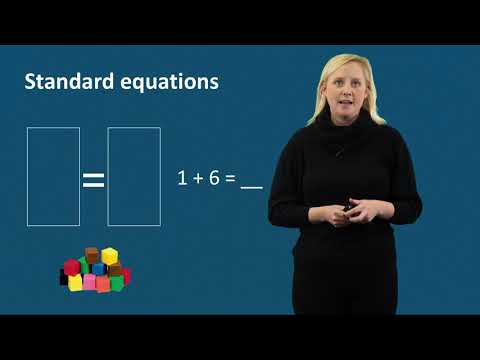### Submit a Resource

Pirate Math Equation QuestPirate Math Equation Quest is an intensive word-problem intervention for elementary children.

The site has downloadable books and instructional videos for teachers and students with a focus on individual and small group intervention.

Pirate Math Equation Quest was developed as an extension of the Pirate Math program developed by Lynn Fuchs, Ph.D., and her colleagues at Vanderbilt University.

From 2015 to 2019, they provided third-grade students experiencing mathematics difficulty with an intensive word-problem intervention called Pirate Math Equation Quest. During Pirate Math Equation Quest, students received explicit instruction on reading, interpreting, setting up, and solving word problems with a focus on schemas. Students also received modeling and practice related to understanding the equal sign and equation solving within the word-problem intervention.

They identified eligible students experiencing mathematics difficulty as those scoring at or below the 13th percentile on a measure of word problems. During 2015-2018, the first three cohorts of students in Pirate Math Equation Quest: Individual Word-Problem Intervention With Total, Difference, and Change Schemas received the word-problem intervention 3 times per week, for 16 weeks, with sessions lasting approximately 30 minutes.

With the final cohort of students in 2018-2019, they introduced Pirate Math Equation Quest: Small-Group Word-Problem Intervention With Total, Difference, Change, and Equal Groups Schemas as a small-group word-problem intervention in which groups of 3–4 students received the word-problem intervention 3 times per week, for 13 weeks, with sessions lasting approximately 30 minutes. The final cohort of students also received a small-group intervention focused on understanding word problems in the State of Texas Assessments of Academic Readiness (STAAR). Students in Pirate Math Equation Quest: Small-Group Intervention for Texas Word Problems in the Grade 3 State of Texas Assessments of Academic Readiness (STAAR) received word-problem intervention 3 times per week, for 6 weeks, with sessions lasting approximately 30 minutes.

Rigorous evaluations of all three iterations of Pirate Math Equation Quest indicated that third-grade students experiencing mathematics difficulty demonstrated improved word-problem performance with Pirate Math Equation Quest compared to students who did not participate in the intervention (Powell, Berry, & Barnes, 2019).

K-6, Educator

## Organization

Pirate Math Equation Quest

Lesson Plan
PDF File